Quadratic Equations - Complete the Square

Introduction by Example

One way to deal with quadratic equations is completing the square, where one takes an equation that does not quite resolve into a nice squared linear factor, such asThe method simply states that one makes it look like a perfect square on one side by adding an appropriate constant to both sides (in this case add 1 to both sides)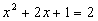This equation is then factored in a straightforward way,and the solutions are found by simply taking the square root of both sides:Clearly these would be difficult to find by guessing. Also, note that there is a +/- symbol, indicating that there are two roots, one using the + sign and the other using the – sign. This is typical of quadratics. Since a quadratic is also known as a second-degree polynomial, it can have at most two distinct roots (which do not have to be real numbers, more on this later).

General Complete the Squares Method

To find the general method of completing the square, note that we can always start with an equation like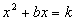Sincewe should examine the square termSubtracting yields a difference ofTherefore, adding this term to both sides of our beginning equation above yieldsor,Example 1

Solve the following equation for xSolution

Here b = 16, b/2 = 8 and k = -8 like so:Plugging these numbers into our formula above givesorback to examples

Example 2

Solve the following equation for xSolution

First, divide the entire equation by 9:Now, b = -4, b/2 = -2 and k = 3, so plugging this into the formula givesback to examples

Example 3

Solve for xSolution

Multiply by 2 to get rid of the coefficient in front of the first termthen subtract 4 from both sides to put the equation in a form as aboveNow it is apparent that b = 4, b/2 = 2 and k = -4. Plug these into the complete the square formula to getIn other words,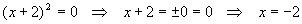This equation has only one repeated root. Remember that quadratics have at most two distinct roots.

back to examples

Example 4

Solve for x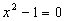Solution

Even though this equation can be solved by simpler means, we are looking at using the complete the square formula, so add 1 to both sides and put in a "ghost" or zero term to hold the "x" place: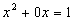Now that the equation is in standard form, we have b = 0, b/2 = 0 and k = 1. Plugging these values into the equation yieldsThis is as expected, and verifies that complete the square agrees with common sense.

back to examples

Example 5

Solve for xSolution

Here b = -2, b/2 = -1 and k = -5. Plugging these in givesso thatandThis solution is interesting in that the two solutions are complex conjugates.

back to examples

Example 6

Solve for xSolution

This problem is best solved by a substitution: let u = x + 2 so that x = u – 2. Putting these into either side of the equation yieldsThis puts the equation in standard form with b = -1, b/2 = -1/2 and k = -4, so, completing the square,A little algebra yieldsDon’t forget that we need to find x, not u, so we have one more step to go:While this method might at first seem a bit complicated, it has the advantage of being very clean, avoids multiplying out a quadratic term and combining with the rest of the equation, then putting the whole thing into standard form. Substitutions like this become very important in more advanced math, and are well worth learning. Normally, they really do simplify calculations, and also often allow them to be checked for errors much more easily.

back to examples